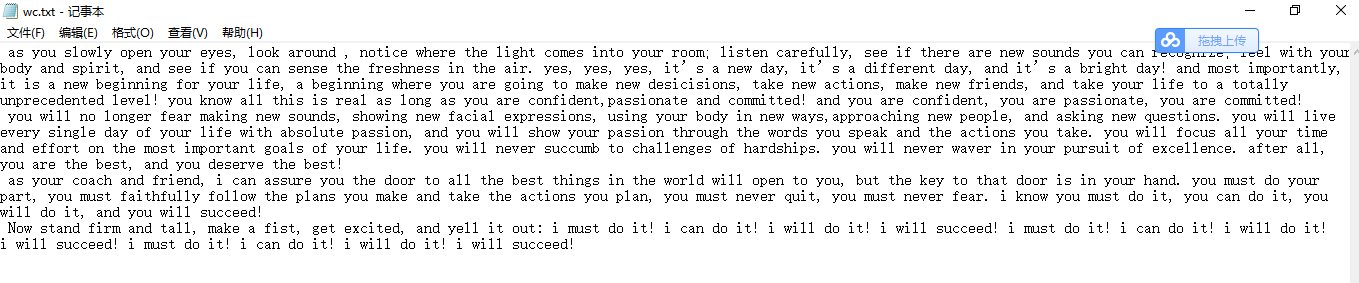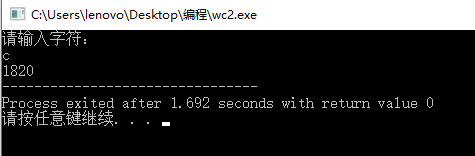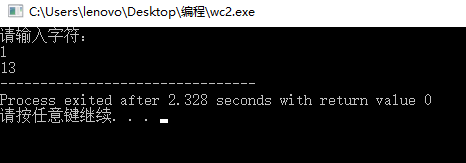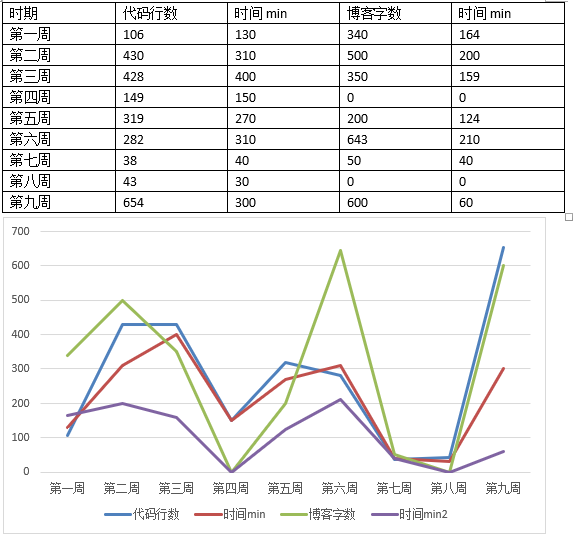# C程序第四次作业

## 作业要求一

#include <stdio.h>
#include <process.h>
int main(int argc,char *argv[])
{
FILE *fp;
int number;
char a;
printf("请输入字符：\n");
scanf("%c",&a);
if(a=='c') {
number=ch();
} else if(a=='w') {
number=wo();
} else {
number=li();
}
printf("%d",number);
return 0;
}
int ch() {
FILE *fp;
if((fp=fopen("wc.txt","r"))==NULL)
{
printf("File to open error!\n");
exit(-1);
}
int m=0;
char a;
a = fgetc(fp);//
while(a!=EOF) {
m++;
a=fgetc(fp);
}
if( fclose(fp) )
{
printf( "Can Not Close The File!\n" );
return 0;
}
return m;
}
int wo () {
FILE *fp;
if((fp=fopen("wc.txt","r"))==NULL)
{
printf("File to open error!\n");
exit(-1);
}
char b;
int i=1,count=0;
b = fgetc(fp);
while(b!=EOF) {
if(b==' '||b=='\n')
{
i=1;
}else
{
if(i==1)
{
count++;
i=0;
}
}
b = fgetc(fp);
}
if( fclose(fp) )
{
printf( "Can Not Close The File!\n" );
return 0;
}
return count;
}
int li() {
FILE *fp;
if((fp=fopen("wc.txt","r"))==NULL)
{
printf("File to open error!\n");
exit(-1);
}
int n=1;
char c;
c= fgetc(fp);
while(c!=EOF) {
if(c=='\n') {
n++;
}
c= fgetc(fp);
}
if( fclose(fp) )
{
printf( "Can Not Close The File!\n" );
return 0;
}
return n;
}## 要求二、学习总结和进度

### 1、将PTA作业的源代码使用git提交到托管平台上，要求给出上传成功截图和你的git地址。git地址:(https://git.coding.net/exo07/disizhouzuoye.git)

### 3、请用表格和折线图呈现你本学期的代码行数和所用时间、博客字数和所用时间，包括ACM刷题等其他平时自己练习的代码### 4、本学期课程即将结束，请总结下你本课程的收获，回顾第0次作业，你是否实现了你的目标？

a:在指针方面自己学到了如何去定义指针变量、指针变量的引用、用指针指向数组且进行相应的运算、指针的指向各种类型（指针，字符串，结构体等等），自我感觉前三点掌握的还算熟练以外其他都是需要多加磨炼的。
b:在结构体方面学到了结构体变量的引用和初始化，及结构体数组的应用，感觉这章的知识点较少，因此自己的掌握程度较熟练。
c:在链表这章，自己主要学习到了链表的定义即如何去创建链表及输出链表，而在链表的插入及删除方面属于懂得原理，但实际操作起来的话会需要时间的程度。
d:在文件方面学到了如何去打开和关闭文件，了解了文件的读和写。

### 6、终于我们即将不再是师生。当你结束本课程，你和我就站在同样的位置上，我将不再基于学校授权和知识、经验 (年龄？)上的优势而对你的自由有任何干扰。除发布成绩以外，我最后一次行使教师特权: 请问你有什么要对教师 (我)说的，建议、抱怨、希望……多谢。

posted @ 2018-05-04 20:01  董雅洁  阅读(273)  评论(10编辑  收藏  举报